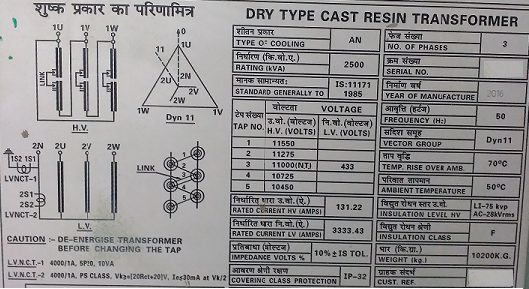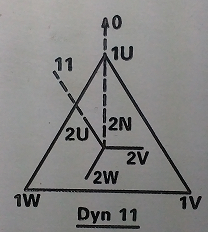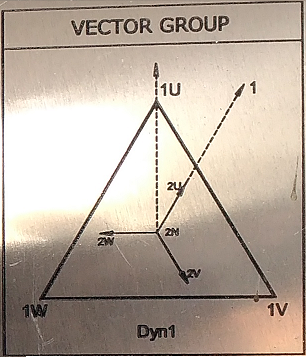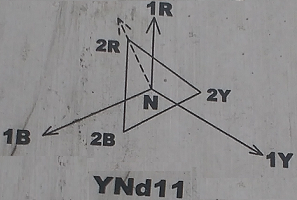# Vector Groups of Transformer:

## PHASOR/VECTOR GROUPS:

Phasor or Vector groups are used to denote different connection methods for poly phase transformers. This knowledge is required for connecting the poly phase transformers in parallel.

To understand the designation of these groups one has to know the assumptions.
These are:
1. In the phasor diagrams, the phasors rotate in the counter clockwise direction.
2. We use Clock Method of Angle Designation
According to this method, the HV line phasor is considered as the minute hand always set at 12 O’clock (zero hour) position and the corresponding LV line phasor is represented by hour hand.

As we know that each digit in clock differs by adjacent one with 30°.
For ex: Take Yd11
Here capital letter(Y) denotes HV winding, small letter (d) denotes LV winding and NUMBER (11) denotes phase displacement between HV and LV emfs expressed as clock hour number.
It represents a poly phase transformer with HV winding in star, LV winding in delta and the LV line phasor 11 O’clock i.e 30° ahead of the zero hour position of the HV line phasors.

## Different Phasor Groups:

Group-1 (0° phase displacement)
• In this connection both HV and LV phasors are in phase with each other.
• In this group Yy0 and Dd0 connections are available.
• Here zero denotes both minute and hour hands are in same position i.e. at 12 O’ clock.
• LV phasors are inphase with the HV phasors .

Group-2 (180° phase displacement)
• In this connection both HV and LV phasors are at 180° phase displacement with each other.
• In this group Yy6 and Dd6 connections are available.
• Here 6 denotes minute hand is on 12 O’ clock and hour hand is on 6 O’ clock.
• LV phasors lead the HV phasors by 180°.

Group-3 (-30° phase displacement)
• In this connection both HV and LV phasors are at 30° phase displacement with each other.
• In this group Yy1 and Dd1 connections are available.
• Here 1 denotes minute hand is at 12 O’ clock and hour hand is at 1 O’ clock.
• LV phasors lag the HV phasors by 30°.

Group-4 (+30° phase displacement)
• In this connection both HV and LV phasors are at 30° phase displacement with each other.
• In this group Yy11 and Dd11 connections are available.
• Here 11 denotes minute hand is at 12 O’ clock and hour hand is at 11 O’ clock.
• LV phasors lead the HV phasors by 30°.

The real time examples for Phasor Groups of transformer are given here. These Phasor Groups can be better understand by the following examples. The Vector diagrams are given in the name plate details of the transformer.

## Examples of Vector Groups:

Every Transformer Contains its own Vector Group as shown below figure.Transformer Name Plate

The following are the mostly used Vector groups of transformers.

Example-1:

As shown in the figure the Vector Group is Dyn11Dyn11 Vector Group

The following information is obtained from the Vector diagram.

• The primary side is Delta denoted by D
• The secondary side is Star with Neutral Connection denoted by yn
• NUMBER (11) denotes phase displacement between HV and LV emfs expressed as clock hour number.

Here Dyn11 represents

A poly phase transformer with HV winding in Delta, LV winding in star with neutral and the LV line phasor 11 O’clock i.e. 30° ahead of the zero hour position of the HV line phasors.

As shown in the above figure the transformer is having 3 phases on HV Side (1U,1V,1W) and 3 Phases on LV Side (2U,2V,2W).

It means the LV Side U phase Vector 2U leads the HV Side U phase Vector 1U by 30°.

It is applicable to other phases also.

LV Side V phase Vector 2V leads the HV Side V phase Vector 1V by 30°.

LV Side W phase Vector 2W leads the HV Side W phase Vector 1W by 30°.

Example-2:

As shown in the figure the Vector Group is Dyn1Dyn1 Vector Group

The following information is obtained from the Vector diagram.

• The primary side is Delta denoted by D
• The secondary side is Star with Neutral Connection denoted by yn
• NUMBER (1) denotes phase displacement between HV and LV emfs expressed as clock hour number.

Here Dyn1 represents

A poly phase transformer with HV winding in Delta, LV winding in star with neural and the LV line phasor 1 O’clock i.e. 30° behind of the zero hour position of the HV line phasors.

As shown in the above figure the transformer is having 3 phases on HV Side (1U,1V,1W) and 3 Phases on LV Side (2U,2V,2W).

It means the LV Side U phase Vector 2U lags the HV Side U phase Vector 1U by 30°.

It is applicable to other phases also.

LV Side V phase Vector 2V lags the HV Side V phase Vector 1V by 30°.

LV Side W phase Vector 2W lags the HV Side W phase Vector 1W by 30°.

Example-3:

As shown in the figure the Vector Group is YNd11.YNd11 Vector Group

The following information is obtained from the Vector diagram.

• The primary side is Star with Neutral Connection denoted by YN
• The secondary side is Delta Connection denoted by d
• NUMBER (11) denotes phase displacement between HV and LV emfs expressed as clock hour number.

Here YNd11 represents

A poly phase transformer with HV winding in Star with neutal, LV winding in delta and the LV line phasor 11 O’clock i.e. 30° ahead of the zero hour position of the HV line phasors.

As shown in the above figure the transformer is having 3 phases on HV Side (1R,1Y,1B) and 3 Phases on LV Side (2R,2Y,2B).

It means the LV Side R phase Vector 2R leads the HV Side R phase Vector 1R by 30°.

It is applicable to other phases also.

LV Side Y phase Vector 2Y leads the HV Side Y phase Vector 1Y by 30°.

LV Side B phase Vector 2B leads the HV Side B phase Vector 1B by 30°.